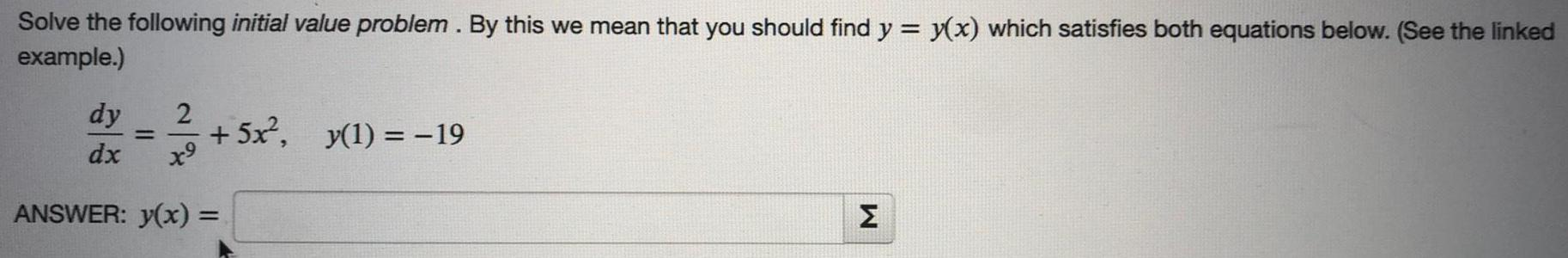Question:

# Solve the following initial value problem. By this we meanSolve the following initial value problem. By this we mean that you should find y = y(x) which satisfies both equations below. (See the linked example.) 2 = 13/03 +5x², _y(1) = −19 x⁹ ANSWER: y(x) = dy dx M